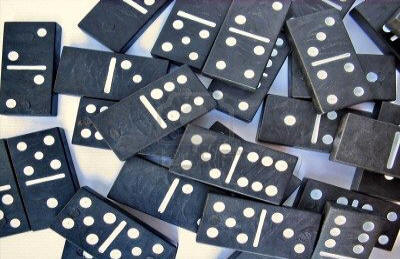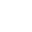Game Explained
 Barnard Castle and District 5s & 3s LeagueRules | Contact Details | Game Explained

What is Fives And Threes?Fives and Threes is a dominoes game for up to four players. The object is to lay your dominoes so that the open ends of the line of dominoes add up to multiples of three and/or five.

Rules

There are many variations on the basic game, but these are the rules used by Fives and Threes in the Teesdale League.

1. There are four or two players.
2. A Cribbage board is normally used to keep score.
3. Each player draws six dominoes if there are four players, if only two then nine.
4. A draw should be made to determine which player starts, usually the highest domino will begin. Any domino can be used to begin
5. Each player now takes it in turn to add a domino to either end of the line of dominoes, so that the numbers on the adjacent ends are the same. If a player cannot play a domino, then he "knocks", so the other players know, and then misses his turn.
6. If the sum of the exposed ends of the line of dominoes adds up to a multiple of five and/or three then the player scores a number of points based on that multiple, or a single three or five counts as one point. Doubles are laid across the normal line of play so that both numbers are considered exposed.
For example, if the exposed ends on the line of dominoes added up to 6, then the player scores 2 points since 6 = 2 x 3. If the exposed ends add up to 12 then the player scores 4 points, since 12 = 4 x 3. If the exposed ends add up to 15 then the player scores 8 points, since 15 = 5 x 3 and 15 = 3 x 5.
7. Play continues until a player has used all his dominoes, or no player can lay any more dominoes.
8. The dominoes are then shuffled and each player draws six for the next round.
9. The game continues until a player reaches 61 exactly. If a player is on 60 points and scores two points, then those points don't count. He has to gain one point to finish on exactly 61.
10. The winning point(s) cannot be scored on a players down